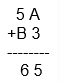# If 5 A + B 3 = 65,

Question:

If 5 A + B 3 = 65, then the value of A and B is

(a) A = 2, B = 3

(b) A = 3, B = 2

(c) A = 2, B = 1

(d) A = 1, B = 2

Solution:

The correct answer is option (c) A = 2, B = 1

Explanation:In the 1’s column A +3 = 5

When A is added with 3, it gives 5

Since A is a digit, it should be between 0 and 9

When you substitute A = 2, you will get 2+3 = 5

Similarly, repeat the process for 10’s column

Then we will get B= 1

Therefore A= 2, and B = 1